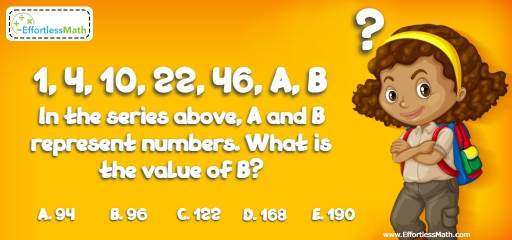# Intelligent Math Puzzle – Challenge 78

This is a great and interactive math puzzle that requires some logic and critical thinking skills to be solved. The answer is given.## Challenge:

1, 4, 10, 22, 46, A, B
In the series above, A and B represent numbers. What is the value of B?

A- 94

B- 96

C- 122

D- 168

E- 190

### The Absolute Best Book to Challenge Your Smart Student!

To the value of B, you should find the pattern of the numbers.
The difference of the first number, 1, and second number, 4, is 3. The difference of 4 and 10 is 6. The difference of 10 and 22 is 12 and the difference of 22 and 46 is 24.
The differences are:
3, 6, 12, 24,
Therefore, the difference of each item is twice the difference of the previous item and the number before that. So, the difference of number A and 46 is 48.
A = 46 + 48 = 94
The difference of B and 94 is 96 (2 × 48).
B = 94 + 96 = 190

The Best Books to Ace Algebra

### What people say about "Intelligent Math Puzzle – Challenge 78 - Effortless Math: We Help Students Learn to LOVE Mathematics"?

No one replied yet.

X
45% OFF

Limited time only!

Save Over 45%

SAVE $40 It was$89.99 now it is \$49.99